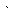# MINLPLib

### A Library of Mixed-Integer and Continuous Nonlinear Programming Instances

#### Instance: flay02m

`Determine the optimal length and width of a number of rectangular patches of land with fixed area, such that the perimeter of the set of patches is minimized.`
 Formatsⓘ ams gms mod nl osil Primal Boundsⓘ 37.94733192 p1 ( gdx sol ) (infeas: 0) Dual Boundsⓘ 37.94625900 (ALPHAECP)37.94733100 (ANTIGONE)37.94733169 (BARON)37.94733192 (BONMIN)37.94733192 (COUENNE)37.94733192 (LINDO)37.94733035 (SCIP) Referencesⓘ Sawaya, Nicolas W, Reformulations, relaxations and cutting planes for generalized disjunctive programming, PhD thesis, Carnegie Mellon University, 2006. Sourceⓘ FLay02M.gms from CMU-IBM MINLP solver project page Applicationⓘ Layout Added to libraryⓘ 28 Sep 2013 Problem typeⓘ MBNLP #Variablesⓘ 14 #Binary Variablesⓘ 4 #Integer Variablesⓘ 0 #Nonlinear Variablesⓘ 2 #Nonlinear Binary Variablesⓘ 0 #Nonlinear Integer Variablesⓘ 0 Objective Senseⓘ min Objective typeⓘ linear Objective curvatureⓘ linear #Nonzeros in Objectiveⓘ 2 #Nonlinear Nonzeros in Objectiveⓘ 0 #Constraintsⓘ 11 #Linear Constraintsⓘ 9 #Quadratic Constraintsⓘ 0 #Polynomial Constraintsⓘ 0 #Signomial Constraintsⓘ 2 #General Nonlinear Constraintsⓘ 0 Operands in Gen. Nonlin. Functionsⓘ Constraints curvatureⓘ convex #Nonzeros in Jacobianⓘ 36 #Nonlinear Nonzeros in Jacobianⓘ 2 #Nonzeros in (Upper-Left) Hessian of Lagrangianⓘ 2 #Nonzeros in Diagonal of Hessian of Lagrangianⓘ 2 #Blocks in Hessian of Lagrangianⓘ 2 Minimal blocksize in Hessian of Lagrangianⓘ 1 Maximal blocksize in Hessian of Lagrangianⓘ 1 Average blocksize in Hessian of Lagrangianⓘ 1.0 #Semicontinuitiesⓘ 0 #Nonlinear Semicontinuitiesⓘ 0 #SOS type 1ⓘ 0 #SOS type 2ⓘ 0 Infeasibility of initial pointⓘ 49 Sparsity JacobianⓘSparsity Hessian of Lagrangianⓘ```\$offlisting
*
*  Equation counts
*      Total        E        G        L        N        X        C        B
*         12        2        4        6        0        0        0        0
*
*  Variable counts
*                   x        b        i      s1s      s2s       sc       si
*      Total     cont   binary  integer     sos1     sos2    scont     sint
*         15       11        4        0        0        0        0        0
*  FX      0
*
*  Nonzero counts
*      Total    const       NL      DLL
*         39       37        2        0
*
*  Solve m using MINLP minimizing objvar;

Variables  x1,x2,x3,x4,x5,x6,x7,x8,x9,x10,b11,b12,b13,b14,objvar;

Positive Variables  x1,x2,x3,x4,x9,x10;

Binary Variables  b11,b12,b13,b14;

Equations  e1,e2,e3,e4,e5,e6,e7,e8,e9,e10,e11,e12;

e1..  - 2*x9 - 2*x10 + objvar =E= 0;

e2..  - x1 - x5 + x9 =G= 0;

e3..  - x2 - x6 + x9 =G= 0;

e4..  - x3 - x7 + x10 =G= 0;

e5..  - x4 - x8 + x10 =G= 0;

e6.. 40/x7 - x5 =L= 0;

e7.. 50/x8 - x6 =L= 0;

e8..    x1 - x2 + x5 + 69*b11 =L= 69;

e9..  - x1 + x2 + x6 + 79*b12 =L= 79;

e10..    x3 - x4 + x7 + 69*b13 =L= 69;

e11..  - x3 + x4 + x8 + 79*b14 =L= 79;

e12..    b11 + b12 + b13 + b14 =E= 1;

* set non-default bounds
x1.up = 29;
x2.up = 29;
x3.up = 29;
x4.up = 29;
x5.lo = 1; x5.up = 40;
x6.lo = 1; x6.up = 50;
x7.lo = 1; x7.up = 40;
x8.lo = 1; x8.up = 50;
x9.up = 30;
x10.up = 30;

Model m / all /;

m.limrow=0; m.limcol=0;
m.tolproj=0.0;

\$if NOT '%gams.u1%' == '' \$include '%gams.u1%'

\$if not set MINLP \$set MINLP MINLP
Solve m using %MINLP% minimizing objvar;

```

Last updated: 2019-02-14 Git hash: a71254dc